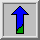# Point Operations# Contents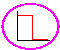Thresholding - select pixels with given values to produce binary imageAdaptive Thresholding - like Thresholding except choose values locally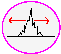Contrast Stretching - spreading out graylevel distribution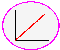Histogram Equalization - general method of modifying intensity distribution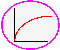Logarithm Operator - reducing contrast of brighter regions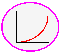Exponential/`Raise to Power' Operator - enhancing contrast of brighter regionsSingle-point processing is a simple method ofimage enhancement. This technique determines a pixel value in the enhanced image dependent only on the value of the corresponding pixel in the input image. The process can be described with themapping function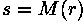where r and s are the pixel values in the input and output images, respectively. The form of the mapping function M determines the effect of the operation. It can be previously defined in an ad-hoc manner, as for thresholding or gamma correction, or it can be computed from the input image, as for histogram equalization. For example, a simple mapping function is defined by the thresholding operator: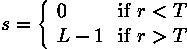The corresponding graph is shown in Figure 1.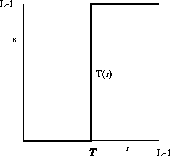Figure 1 Graylevel transformation function for thresholding.

Point operators are also known asLUT-transformations, because the mapping function, in the case of a discrete image, can be implemented in a look-up table (LUT).

A subgroup of the point processors is the set ofanamorphosis operators. This notion describes all point operators with a strictly increasing or decreasing mapping function. Examples include the logarithm operator, exponential operator and contrast stretching, to name just a few.

An operator where M changes over the image is adaptive thresholding. This is not a pure point operation anymore, because the mapping function, and therefore the output pixel value, depends on the local neighborhood of a pixel.